# For the given state of stress, determine (a) the principal planes, (b) the principal stresses.

Question-AnswerCategory: Strength of MaterialsFor the given state of stress, determine (a) the principal planes, (b) the principal stresses.

For the given state of stress, determine (a) the principal planes, (b) the principal stresses.Step: 1

Draw the Schematic diagram of element: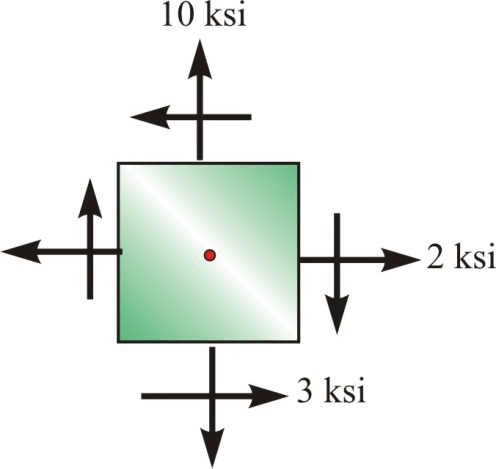Join

Step: 2

(a)
Calculate the orientation of the principal planes by using the following equation: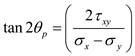Substitute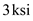for.Therefore, the Principal planes are.

Step: 3

(b)
Calculate the maximum principal stresses by using the following equation:Substitute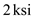forfor, andfor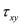.Step: 4

Calculate the minimum principal stresses by using the following equation: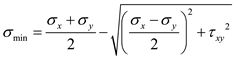Substitutefor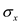for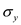, andfor.Therefore, the maximum principal stress is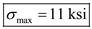and the minimum principal stress is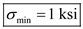.